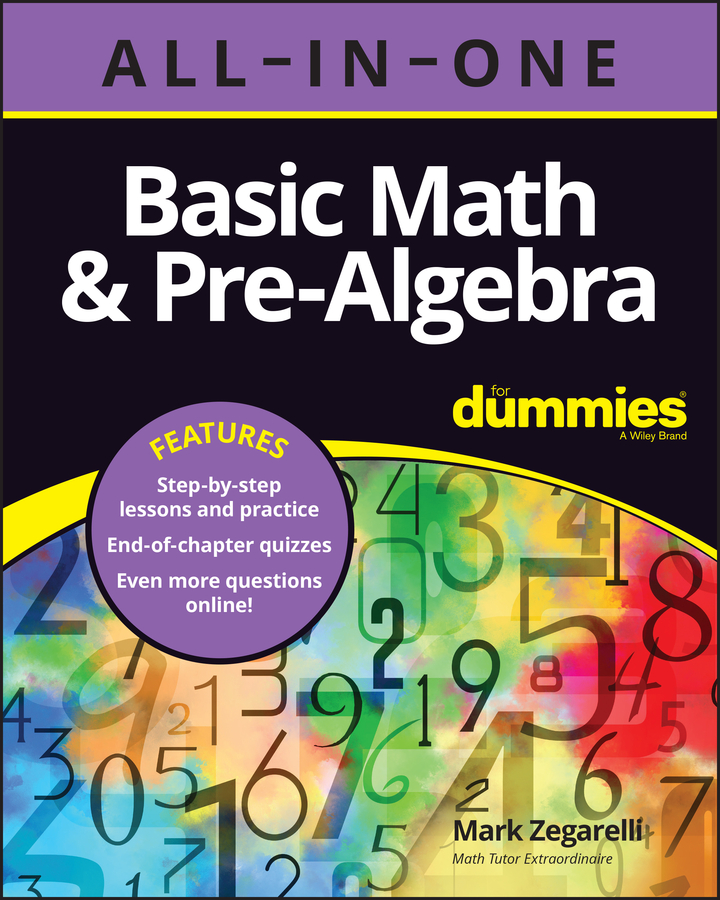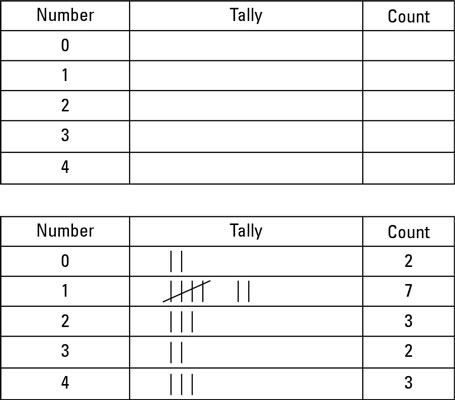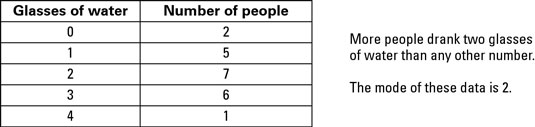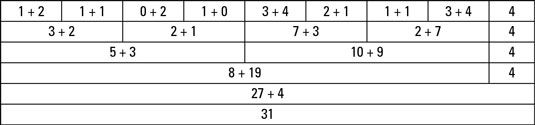##### Basic Math & Pre-Algebra All-in-One For Dummies (+ Chapter Quizzes Online)We use three different types of average in maths: the mean, the mode and the median, each of which describes a different ‘normal’ value. The mean is what you get if you share everything equally, the mode is the most common value, and the median is the value in the middle of a set of data.

Here are some more in-depth definitions:

• Median: In a sense, the median is what you normally mean when you say ‘the average man in the street’. The median is the middle-of-the road number – half of the people are above the median and half are below the median. (In America, it’s literally the middle of the road: Americans call the central reservation of a highway the ‘median’.)

Try remembering ‘medium’ clothes are neither large nor small, but somewhere in between. Goldilocks was a median kind of girl.

• Mode: The mode is the most common result. ‘Mode’ is another word for fashion, so think of it as the most fashionable answer – ‘Everyone’s learning maths this year!’

• Mean: The mean is what you get by adding up all of the numbers and dividing by how many numbers were in the list. Most people think of the mean when they use the word ‘average’ in a mathematical sense.

In some ways the mean is the fairest average –you get the mean if the numbers are all piled together and then distributed equally. But the mean is also the hardest average to work out.

You use the different averages in different situations, depending on what you want to communicate with your sums.

## Find the median

To find the median of a set of numbers, you arrange the numbers into order and then find the number exactly in the middle:
1. If the numbers aren’t in order, sort them out.

You can arrange them either going up or down.

2. Circle the number at each end of the list.

3. Keep circling numbers two at a time (one from each end) until you have only one or two uncircled numbers.

4. If only one number is left, that’s the median.

You’re done!

5. If two numbers are left, find the mean.

Add up the two numbers and divide by two. The answer is the median.

## Find the mode

If you have a list of numbers in order, figuring out which number shows up most often is pretty easy. You simply count the numbers – whichever number you have most of is the mode.

If you have the list 1, 1, 3, 5, 5, 5, 6, 6, 7, 8, 9, 9, 10, you count each number in turn and find you have two 1s, one 3, three 5s, two 6s, one 7, one 8, two 9s and a 10. The number 5 comes up more frequently than any of the others, so the mode of these data is 5.

If the data aren’t in a list, I suggest you set up a tally chart to help you count the numbers.Finding the mode in a table of numbers is very easy: whoever made the table has already done the tally chart for you and counted up the 1s. All you do is find the biggest number in the ‘count’ or ‘frequency’ column. The number labelling that row is the mode.## Working out the mean of a list of numbers

Here’s how to work out the mean of a set of numbers:
1. Write out a list of all the numbers.

2. Add up all the numbers.

3. Count how many numbers are in the list.

4. Divide the total from Step 2 by the total in Step 3.

The answer is the mean.